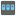• # Why is the test failing on Check iO?

## Question related to missionHouse Password

I would like to give some feedback about ...

HTTP\_USER\_AGENT:

Mozilla/5.0 (X11; Linux x86_64) AppleWebKit/537.36 (KHTML, like Gecko) Ubuntu Chromium/34.0.1847.116 Chrome/34.0.1847.116 Safari/537.36

My Code:

def checkio(data):
if type(data) == str:
if len(data) < 10:
return False

u, l, d = 0, 0, 0
for char in data:
temp = ord(char)
if 65 <= temp <= 90:
u += 1
elif 97 <= temp <= 122:
l += 1
else:
d += 1
if u>=1 and l>=1 and d>=1:
return True
return False

if __name__ == '__main__':
#These "asserts" using only for self-checking and not necessary for auto-testing
assert checkio(u'A1213pokl') == False, "1st example"
assert checkio(u'bAse730onE4') == True, "2nd example"
assert checkio(u'asasasasasasasaas') == False, "3rd example"
assert checkio(u'QWERTYqwerty') == False, "4th example"
assert checkio(u'123456123456') == False, "5th example"
assert checkio(u'QwErTy911poqqqq') == True, "6th example"